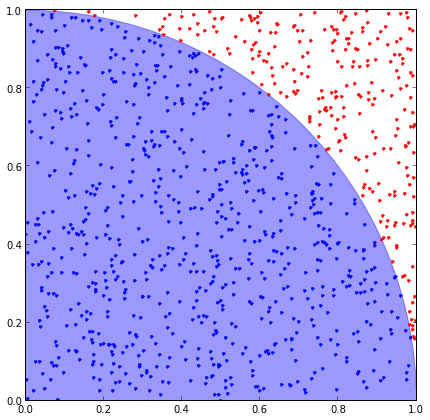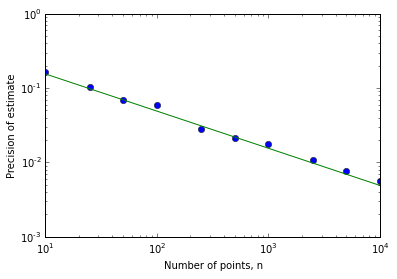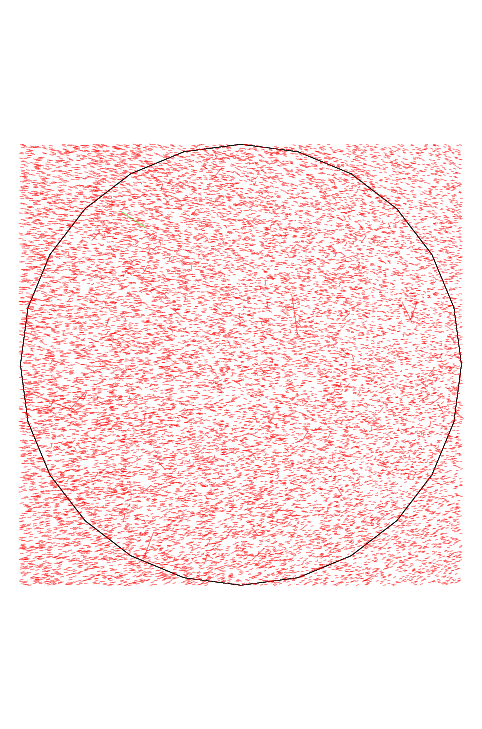# Using random numbers to estimate Pi

I'm a big fan of Monte Carlo methods. There is something neat about performing a complex integration or simulation fueled by an engine running on random numbers.

In this post, I'm going to talk about a canonical example used to demonstrate Monte Carlo integration: estimating the area of the unit circle via hit-or-miss Monte Carlo sampling. Indeed, this toy example is so ubiquitous that it is the focus of the Wikipedia Monte Carlo integration page (I was even asked to describe this problem during my Masters defence).

Here we go:

The unit circle follows the equation $x^2 + y^2 = 1$, and the area of the full circle can be determined from the integral of a quadrent (multiplied by 4 to account for all four quadrents). The area is then expressed as the definite integral,

$A_{\mathrm{circle}} = 4\int_{0}^{1} \sqrt{1-x^2}dx$.

Well, sometimes we just don't feel like doing textbook trigonometric substitutions. In this case we're essentially going to brute force the solution using so-call 'hit-or-miss' Monte Carlo. The procedure is as follows:

1. generate two random numbers, $x$ and $y$, both in the range [0,1]
2. if $x^2 + y^2 < 1$ then add $(x,y)$ to the 'hit' bucket, otherwise add $(x,y)$ to the 'miss' bucket
3. repeat steps 1-2 a total of $n$ times
4. finally, use the fraction of 'hits' to estimate the area of the circles via the formula $4 \frac{\mathrm{hits}}{\mathrm{hits}+\mathrm{misses}}$

The following function uses Python's standard pseudo-random number generator to complete steps 1-3:

In :
import random
def generate_points(n):
'''
Generate n (x,y) points
Return lists of points that fall inside and outside of the unit circle
'''
hits   = []
misses = []

for point in range(n):
x,y = (random.random(), random.random())
hits.append((x,y)) if x*x + y*y <= 1. else misses.append((x,y))
return hits, misses

#here, we'll generate n=1000 x-y points
hits, misses = generate_points(1000)


It is also informative to visualize both the hits and misses. This should hopefully provide a fuller picture of what is going on:

In :
%matplotlib inline
import matplotlib.pyplot as plt

def plot_points(hits,misses):
'''
Plot a unit circle, and the points falling inside and outside
'''
def plot_sets(points,c):
plt.plot([x for x in points],[y for y in points],marker='.',lw=0,c=c)

fig = plt.figure(figsize=(7,7))
plot_sets(hits,'b')
plot_sets(misses,'r')

circle=plt.Circle((0,0),1,color='b', alpha=0.4)
fig.gca().add_artist(circle)

plt.show()

plot_points(hits,misses)Finally, we can summarize the result, estimate the area, and compare tne result to the known value ($\pi$),

In :
from tabulate import tabulate
import math

def estimate(hits,misses):
'''
Estimate the area of the unit circle
'''
return 4.*float(len(hits))/float(len(hits)+len(misses))

def summary(hits,misses):
print
print tabulate([["Number of points inside the circle", len(hits)],
["Number of points outside the circle", len(misses)],
["Estimate of circle area (4*h/(h+m))", estimate(hits,misses)],
["Percent different from pi", round((estimate(hits,misses) - math.pi)/math.pi*100.,1) ]],
tablefmt="plain")

summary(hits,misses)

Number of points inside the circle   7890
Number of points outside the circle  2110
Estimate of circle area (4*h/(h+m))     3.156
Percent different from pi               0.5


With 1000 samples, we get an estimate that is within a couple percent.

## Some notes about convergence¶

It should be pointed out that Monte Carlo integration isn't very fast. In fact, this method converges as $1/\sqrt{n}$, which is to say, if you want a 10% increase in the precision, you need to add 100x more samples. This scaling relationship between the precision of the estimate, and the number of samples is shown here: (where the green line is $\sim1/\sqrt{n}$)

In :
import numpy as np

ns     = []
stdevs = []
sqrts  = []

for n in [10,25,50,100,250,500,1000,2500,5000,10000]:
samples = []
for sample in range(100):
hits, misses = generate_points(n)
samples.append((estimate(hits,misses)-math.pi)/math.pi)
ns.append(n)
stdevs.append(np.std(samples))
sqrts.append(0.5/math.sqrt(n))

plt.loglog(ns,stdevs,'o',ns,sqrts,'-')
plt.xlabel("Number of points, n")
plt.ylabel("Precision of estimate")
plt.show()The $1/\sqrt{n}$ scaling isn't that attractive for 1-D integrations like above (the trapezoid rule, for example, goes as $\sim1/n^2$). However the power of the Monte Carlo method is that the scaling relation holds for higher dimensions, where methods like the trapezoid rule fall down. This is great, as we can still fairly efficiently perform integrations for problems with very large dimensionality.

## Over-the-top misuse of the GEANT4 Monte Carlo simulation package for hit-or-miss Monte Carlo integration¶

Many fields use Monte Carlo methods to simulate physical processes. In particle physics (which is what I'm most familar with), for example, the trajectories of particles are simulated, along with their interactions with materials and other particles, to estimate likely outcomes for experiments. An simulation package used extensively by particle (and medical) physicists is GEANT4.

While GEANT4 is a large and very powerful software package, I'm going to use it here in a somewhat silly way to perform the same hit-or-miss integration of the area of a circle. Essentially, I'm going to simulate an electron being shot at a circular target, where the origin point of the electron is uniformly distributed in an encompassing square (just like above). Then, in the same way, we can count the number of electrons that hit the target, and estimate just how close to $\pi$ we can get!

A note: GEANT4 can be finicky to install. Here I took advantage of a Docker container put together by Simon Biggs, which removed most of the installation hassle and also took advantage of the Python bindings and runs inside an IPython notebook. How cool is that. I've modified Simon's example to my own devious ends:

In :
from Geant4 import *

*************************************************************
Geant4 version Name: geant4-09-06-patch-03    (14-March-2014)
Copyright : Geant4 Collaboration
Reference : NIM A 506 (2003), 250-303
WWW : http://cern.ch/geant4
*************************************************************

Visualization Manager instantiating with verbosity "warnings (3)"...


First off, we'll create the world volume and a thin cylinder target. That will be it for the simulation geometry -- we don't actually want anything to get in the way of the electrons (not even simulated air), because any scattering will cause perturbations in the electron trajectories or even cause the emission of secondary particles.

In :
class MyDetectorConstruction(G4VUserDetectorConstruction):

def __init__(self):

G4VUserDetectorConstruction.__init__(self)

self.solid = {}
self.logical = {}
self.physical = {}

#root volumn
self.create_world()

#target
self.create_target()

def create_world(self):

material = gNistManager.FindOrBuildMaterial("G4_Galactic")
self.solid  ['world'] = G4Box("world", 4000/2., 4000/2., 4000/2.)
self.logical['world'] = G4LogicalVolume(self.solid['world'],
material,
"world")
self.physical['world'] = G4PVPlacement(G4Transform3D(),
self.logical['world'],
"world", None, False, 0)

visual = G4VisAttributes()
visual.SetVisibility(False)

self.logical['world'].SetVisAttributes(visual)

def create_target(self):

translation = G4ThreeVector(0,0,0)
material = gNistManager.FindOrBuildMaterial("G4_Si")
visual = G4VisAttributes(G4Color(1.,1.,1.,0.1))
mother = self.physical["world"]

self.solid["target"] = G4Tubs("target", 0., 1000, 0.0001/2., 0., 2*pi)

self.logical["target"] = G4LogicalVolume(self.solid["target"],
material,
"target")

self.physical["target"] = G4PVPlacement(None, translation,
"target",
self.logical["target"],
mother, False, 0)

self.logical["target"].SetVisAttributes(visual)

def Construct(self): # return the world volume

return self.physical['world']


Here, we define the primary particle emission, which in our case is the electrons. We'll shoot those electrons in the direction $-1\vec{z}$, with energies of 6MeV (which shouldn't really matter in our case). Each iteration, a random starting position is choosen for the electron in GeneratePrimaries.

In :
class MyPrimaryGeneratorAction(G4VUserPrimaryGeneratorAction):

def __init__(self):
G4VUserPrimaryGeneratorAction.__init__(self)

particle_table = G4ParticleTable.GetParticleTable()

electron = particle_table.FindParticle(G4String("e-"))

beam = G4ParticleGun()
beam.SetParticleEnergy(6*MeV)
beam.SetParticleMomentumDirection(G4ThreeVector(0,0,-1))
beam.SetParticleDefinition(electron)
beam.SetParticlePosition(G4ThreeVector(0,0,2000))

self.particleGun = beam

def GeneratePrimaries(self, event):

self.particleGun.SetParticlePosition(
G4ThreeVector(2*(random.random()-0.5)*1000,
2*(random.random()-0.5)*1000,
2000))

self.particleGun.GeneratePrimaryVertex(event)


Here is the sneaky bit. For every step of the simulation, we'll check if the primary electron is inside of the target volume. If yes, we'll increment a hit counter. Please note that this isn't actually a good GEANT4 practice: usually, if you want to check if a particle has traveled through a volume, you'll define a SensitiveDetector, and it'll take care of figuring out if any particles hit that volume. However, since we're already doing naughty things, we'll take this shortcut.

In :
class MySteppingAction(G4UserSteppingAction):

def __init__(self):
G4UserSteppingAction.__init__(self)

self.cnt = 0 #hit counter

def UserSteppingAction(self, step):
track = step.GetTrack()
if track.GetVolume().GetName() == 'target' and track.GetParentID() == 0: #check if the primary electron hit the target
self.cnt = self.cnt+1


Here, we register our user-defined functions with the GEANT4 run manager. We'll use a default Physics List -- event though it defines a lot more processes than we'll actually utilize, it is easier to use a pre-defined list than add all of the processes one-by-one.

In :
gRunManager.SetUserInitialization(FTFP_BERT()) #physics list

detector                 = MyDetectorConstruction()
primary_generator_action = MyPrimaryGeneratorAction()
stepping_action          = MySteppingAction()

gRunManager.SetUserInitialization(detector)
gRunManager.SetUserAction(primary_generator_action)
gRunManager.SetUserAction(stepping_action)
gRunManager.Initialize()

<<< Geant4 Physics List simulation engine: FTFP_BERT 2.0

### Adding tracking cuts for neutron  TimeCut(ns)= 10000  KinEnergyCut(MeV)= 0


The following GEANT4 macro file will define the visualization and define the number of electrons ($n=20000$) used in the simulation:

In :
%%file linac-example/macros/dawn.mac

/vis/open DAWNFILE

/vis/scene/create
/vis/scene/add/volume

/vis/scene/add/trajectories
/vis/scene/endOfEventAction accumulate 2000

/vis/scene/add/hits

/vis/sceneHandler/attach

/vis/viewer/set/targetPoint 0.0 0.0 0.0 mm
/vis/viewer/set/viewpointThetaPhi 0.2 1

/vis/viewer/zoom 1.3

/tracking/verbose 0

/run/beamOn 20000

Overwriting linac-example/macros/dawn.mac


Finally, we'll execute the macro, which will generate an output file with all of the electron trajectory information that we can then visualize.

In :
gUImanager.ExecuteMacroFile('linac-example/macros/dawn.mac')

/tracking/storeTrajectory 1

In :
!mv g4_00.prim images/world.prim
!dawn -d images/world.prim
!convert images/world.eps images/world.png

from IPython.display import Image
Image("images/world.png")


Out:Just like the first section, we see points both inside and outside of the target circle. We can then access the stepping_action hit counter, which recorded the number of electrons that passed through the target volume:

In :
stepping_action.cnt

Out:
15642

With a couple extra lines, we can reuse the summary code from above to make the circle area estimate and percent error:

In :
G4_n    = 20000
G4_hits = range(15642)
G4_misses = range(G4_n - len(G4_hits))

summary(G4_hits,G4_misses)

Number of points inside the circle   15642
Number of points outside the circle   4358
Estimate of circle area (4*h/(h+m))      3.1284
Percent different from pi               -0.4


Not so bad, for a fairly inappropriate use of the GEANT4 framework. Note that, while we could add more electrons to our samples, using this method we won't end up getting a better estimate of $\pi$. This is mainly because the simulation volume used by GEANT4 isn't actually a circle. You can see in the above figure that it is acutally a regular polygon with n=22 or so. Therefore, adding more samples will actually give us a more precise estimate of the area of that polygon, but a biased estimate of $\pi$ (which is a not-bad example of the difference between accuracy and precision).

I've also hand-waved over a bunch of assumptions about the simulation: like if the electron trajectories are actually expected to be straight lines in this medium (I didn't actually check if G4_Galactic has a mean-free-path), also, I made the target volume very thin to hopefully avoid any scattering or secondary generation within the target itself, but when using a default physics list, you could possible end up with some hadronic interaction or large angle scattering that could double-count some electrons in the target. But ultimately, doing something unusal, even if a bit hand-wavy, is the fun part.